# Quiz: Practical 5 Mathematics and Statistics (1910) Quiz: Practical 5 This Question: 2 pts Independent ra...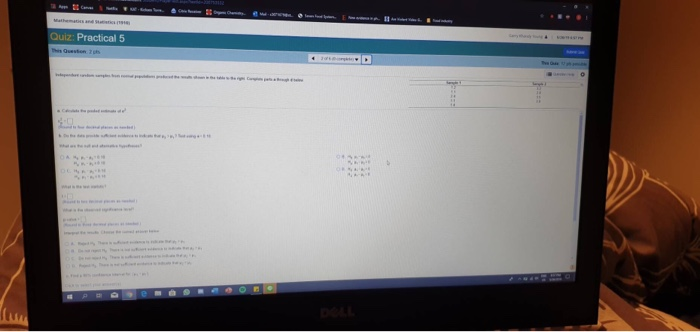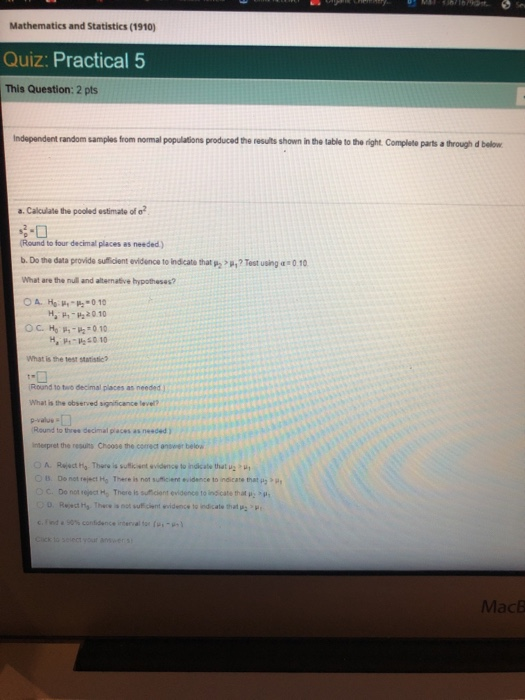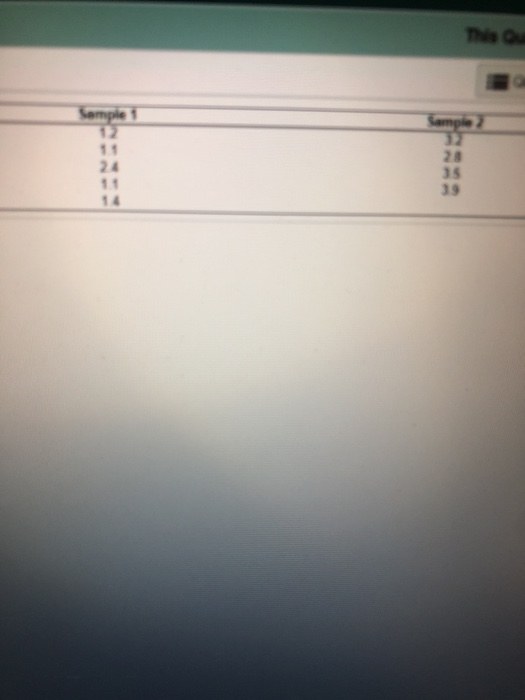Quiz: Practical 5
Mathematics and Statistics (1910) Quiz: Practical 5 This Question: 2 pts Independent random samples from normal populations produced the results shown in the table to the right Complete parts a through d below a. Calculate the pooled estimate of o2 #0 Round to four decimal places as needed) b, Doth° data provide slient evidence toindcato that P2 >р,? Test using .010 What are the null and alternative hypotheses? H, P-20.10 What is the test statistic? Round to two decimal places as needed ) What is the observed significance level? p-value s Round to three decimal places as needed ) interpret the resuits Choose the correct anseer belo A Reject o Thare is sulficiant wvidence to indicate that u B. Donet remet Ha There i, net sumcem evidence to recan" matsa C. Do not rejact There is suicient evidence to indicate t D. Rwet Hg There is not sucint evidence to indicate that MacB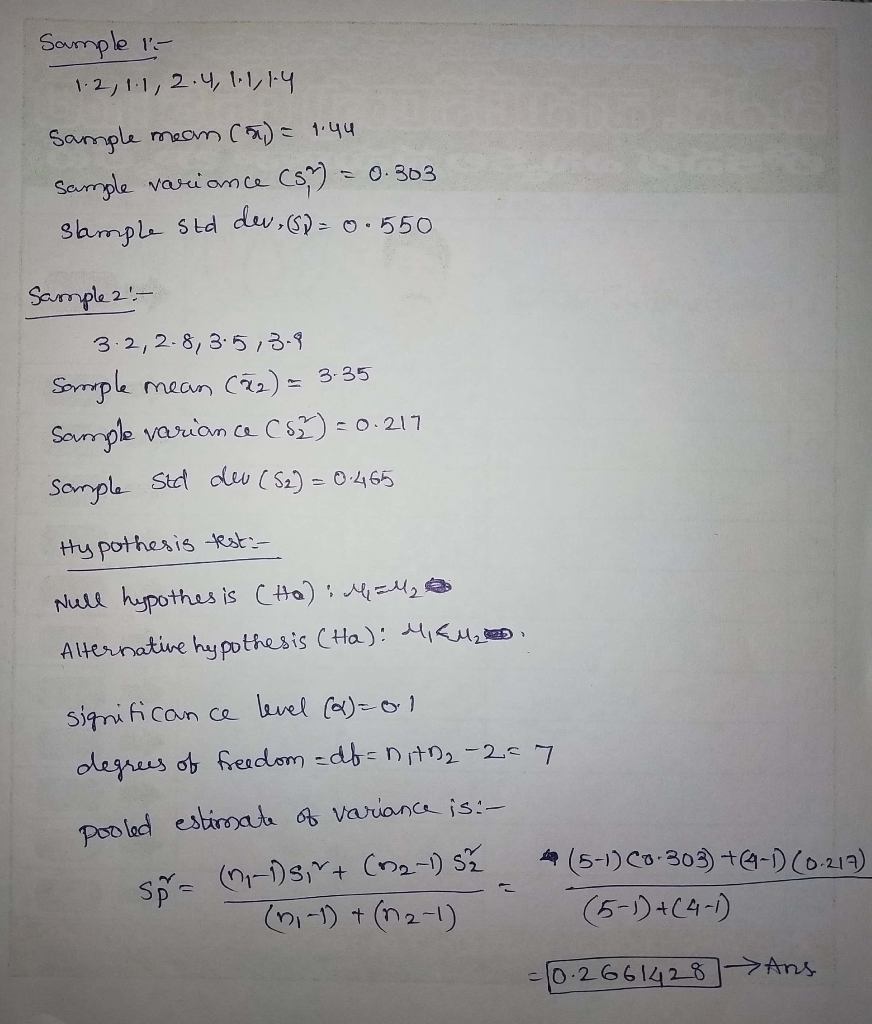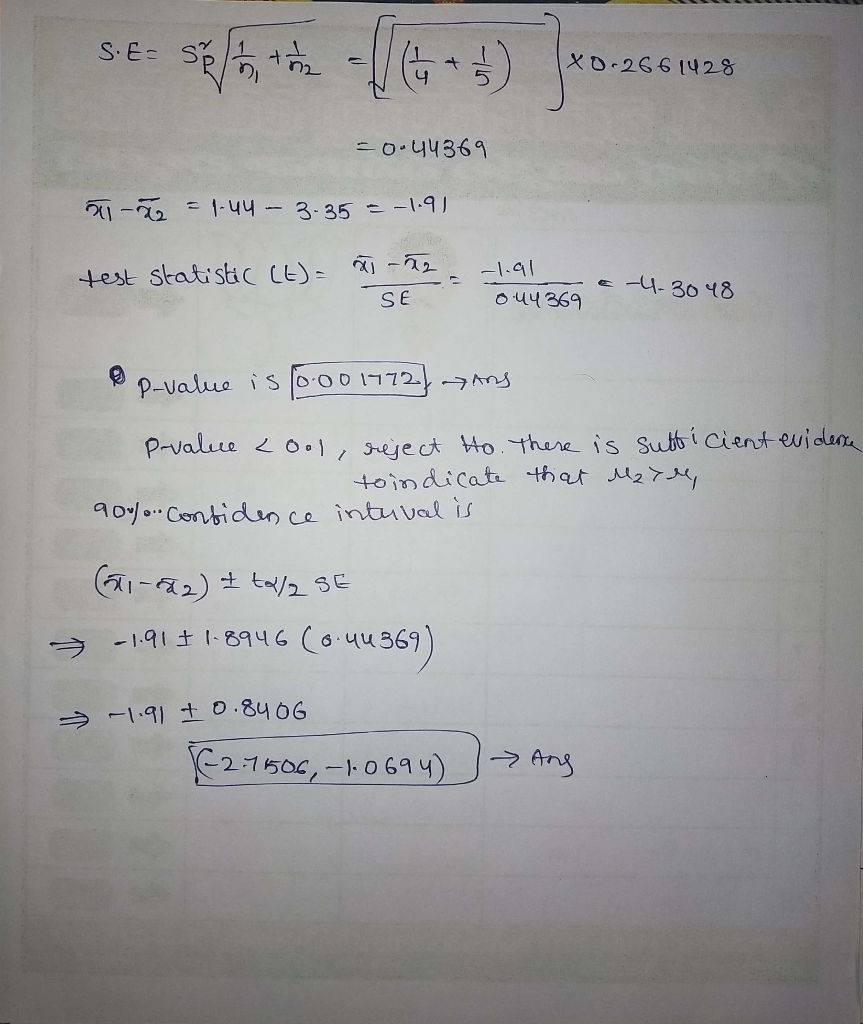##### Add Answer of: Quiz: Practical 5 Mathematics and Statistics (1910) Quiz: Practical 5 This Question: 2 pts Independent ra...
More Homework Help Questions Additional questions in this topic.